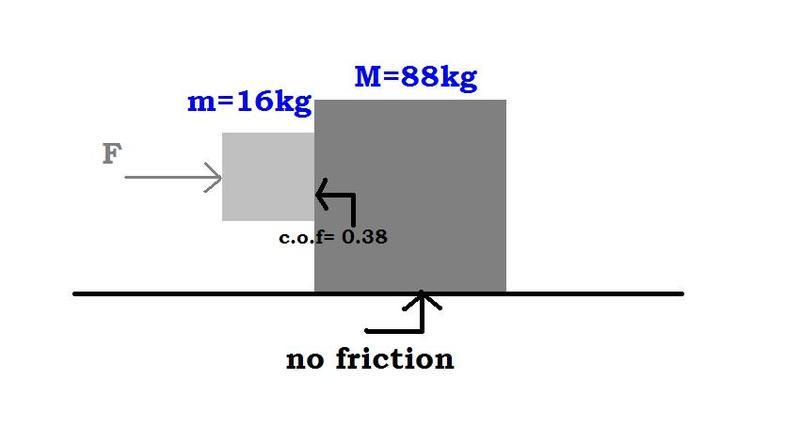# Two blocks held against each other

## Homework StatementTwo blocks m (16kg) and M (88kg) are as shown in the figure. If the co-efficient of friction b/w the blocks is 0.38 but the surface beneath the block is frictionless, what is the minimum force required to hold m against M?

How would you go about solving this kind of a problem? which concept am i required to use?

## Homework Equations

F(friction)= c.o.f * F(normal)

## The Attempt at a Solution

So i tried solving it (its too lengthy to write exactly what i did) but i ended up with a final answer of F = 2.68e3 N. Can someone double check that? thanks

#### Attachments

Last edited:

rl.bhat
Homework Helper
The solution need not be lengthy.
If F is the applied force, what is the common acceleration of M and m?
What is normal reaction on m by M?
What is the frictional force between m and M? What is its direction?
Which force pulls m down ward direction?

How are you supposed to get the system's acceleration though?

Fa = Force Applied
Ff = Force Friction
Fg = Force Gravity
Fn = Force Normal

$$\Sigma$$Fx = MA

Fa = 104a
a = Fa/104?

Doesn't really help unless I'm missing something.

Force causing the friction (gravity) is:

Fg = 16(9.81)
Fg = 157N

Therefore, the block has 157 Newton's being pulled down.

$$\Sigma$$Fy = MA

The block is held still, therefore, no acceleration
$$\Sigma$$Fy = 0
Ff - Fg = 0

$$\mu$$Fn - 157 = 0
Fn = 157/(.38)
Fn = 413N

This would be, in theory, the force that the big 88 Kg block exerts on the small block... But it still doesn't help me find the dang acceleration

Edit: Oh nevermind... ha ha... Yeah it does. I can then use the system of the 2nd block to find the acceleration of the full system.